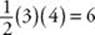﻿ ﻿PERIMETER AND AREA OF QUADRILATERALS - Quadrilaterals and Other Polygons - PLANE GEOMETRY - SAT SUBJECT TEST MATH LEVEL 1

## PLANE GEOMETRY## CHAPTER 10Quadrilaterals and Other Polygons### PERIMETER AND AREA OF QUADRILATERALS

The perimeter (P ) of a polygon is the sum of the lengths of all of its sides. The area (A) of a polygon is the amount of space it encloses (measured in square units). The perimeter and area formulas you need to know for the Math 1 test are given in KEY FACTS I8 and I9.

Key Fact I8

PERIMETERS

For a rectangle: P = 2(+ w)

For a square: P = 4sKey Fact I9

AREAS

For a parallelogram: A = bh

For a rectangle: A =w

For a square: A = s2 orFor a trapezoid:TIPHere”s a useful alternate formula for the area of a square:
If d is the diagonal of a square, then the area of the square is.

EXAMPLE 3: What are the perimeter and area of a rhombus whose diagonals are 6 and 8? First draw and label a rhombus.Since the diagonals bisect each other, BE = ED = 3 and AE = EC = 4. Also, since the diagonals of a rhombus are perpendicular, ∠BEA is a right angle andBEA is a 3-4-5 right triangle. So AB = 5 and the perimeter of the rhombus is 45 = 20. The easiest way to calculate the area of the rhombus is to recognize that it is the sum of the areas of four 3-4-5 right triangles. Since each triangle has an area of, the area of the rhombus is 46 = 24.

EXAMPLE 4: In the figure below, the area of parallelogram ABCD is 40. What are the areas of rectangle AFCE, trapezoid AFCD, and triangle BCF ?Since the base of parallelogram ABCD is 10 and its area is 40, its height, AE, must be 4. Then ΔAED must be a 3-4-5 right triangle with DE = 3, which implies that EC = 7. So the area of rectangle AFCE is 74 = 28; the area of trapezoid AFCD is; and the area of each small triangle is.

﻿